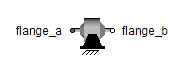Stepper Permanent Magnet - MapleSim Help

Stepper Permanent Magnet

Permanent magnet stepper motorDescription The Stepper Permanent Magnet (or Stepper Motor PM) component models a two-phase permanent magnet stepper machine. The winding resistances and inductances of the two phase windings are identical. The losses in the winding resistances only are taken into account; saturation is not modeled.Equations $J\stackrel{.}{\mathrm{\omega }}+B\mathrm{\omega }=-{K}_{m}{i}_{a}\mathrm{sin}\left(\mathrm{Nr}\mathrm{\theta }\right)+{K}_{m}{i}_{b}\mathrm{cos}\left({N}_{r}\mathrm{\theta }\right)-\mathrm{\tau }$ $L\stackrel{.}{{i}_{a}}+R{i}_{a}={v}_{{a}_{p}}-{v}_{{a}_{n}}+{K}_{m}\mathrm{\omega }\mathrm{sin}\left({N}_{r}\mathrm{\theta }\right)$ $L\stackrel{.}{{i}_{b}}+R{i}_{b}={v}_{{b}_{p}}-{v}_{{b}_{n}}-{K}_{m}\mathrm{\omega }\mathrm{cos}\left({N}_{r}\mathrm{\theta }\right)$ $\mathrm{\omega }=\stackrel{.}{\mathrm{\theta }}$ ${i}_{a}={i}_{{a}_{p}}=-{i}_{{a}_{n}}$ ${i}_{b}={i}_{{b}_{p}}=-{i}_{{b}_{n}}$ $\mathrm{\theta }={\mathrm{\phi }}_{\mathrm{rflange}}$ $\mathrm{\tau }={\mathrm{\tau }}_{\mathrm{rflange}}$Connections

 Name Description Modelica ID ${\mathrm{neg}}_{{\mathrm{pin}}_{a}}$ Negative pin winding a neg_pin_a ${\mathrm{pos}}_{{\mathrm{pin}}_{a}}$ Positive pin winding a pos_pin_a ${\mathrm{neg}}_{{\mathrm{pin}}_{b}}$ Negative pin winding b neg_pin_b ${\mathrm{pos}}_{{\mathrm{pin}}_{b}}$ Positive pin winding b pos_pin_b $\mathrm{rflange}$ Shaft end rflangeParameters

 Name Default Units Description Modelica ID $J$ $0.005$ $\mathrm{kg}{m}^{2}$ Rotor moment of inertia J $B$ $0.08$ $\frac{Nms}{\mathrm{rad}}$ Rotor damping B $R$ $1.55$ $\mathrm{\Omega }$ Winding resistance R $L$ $0.015$ $H$ Winding inductance L ${K}_{m}$ $0.19$ $\frac{Nm}{A}$ Machine torque constant Km ${N}_{r}$ $50$ $1$ Number of rotor teeth Nr